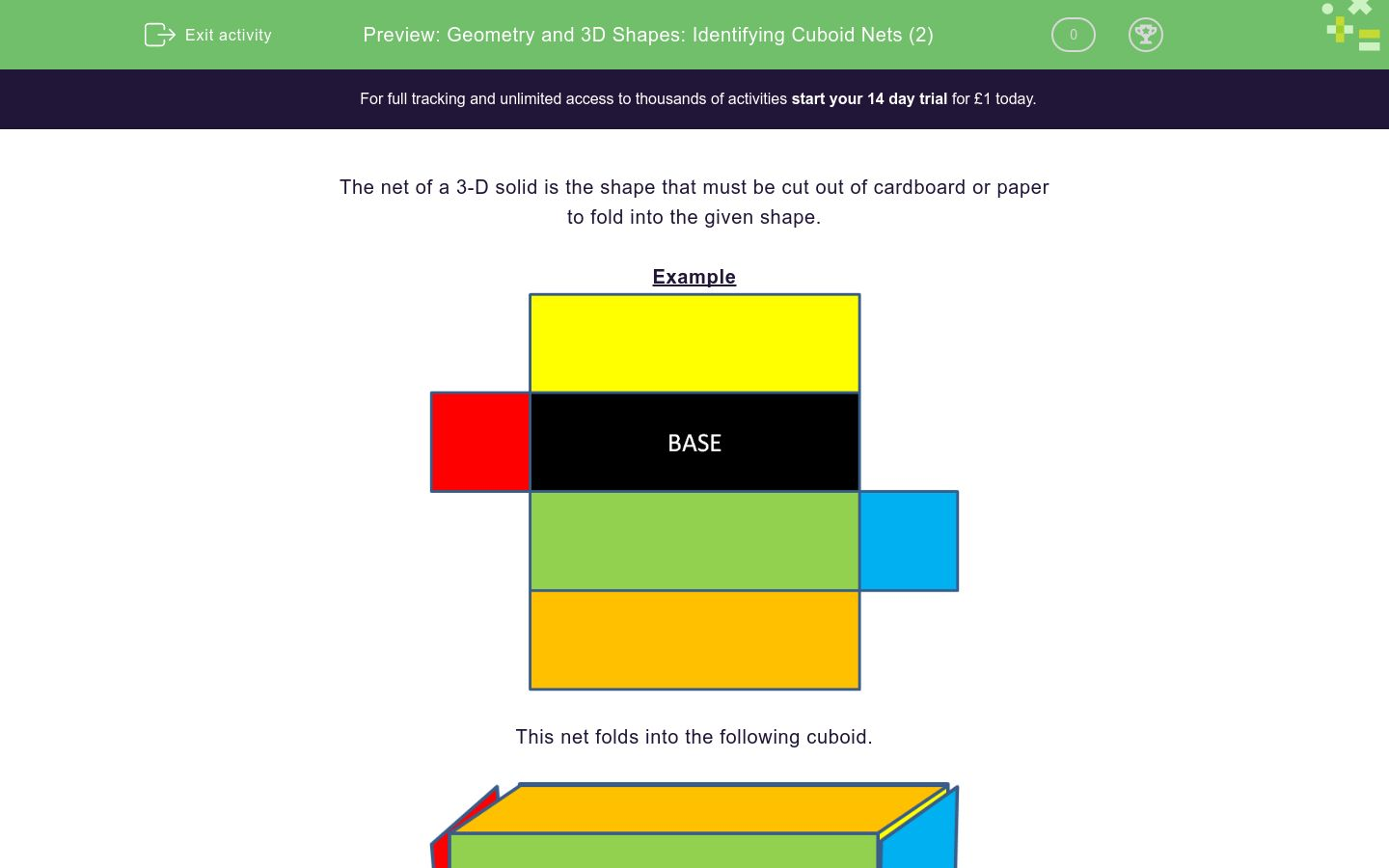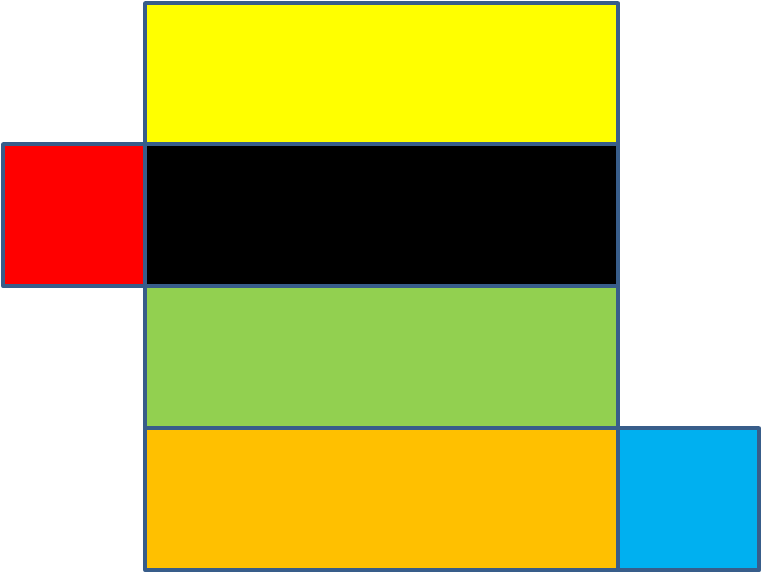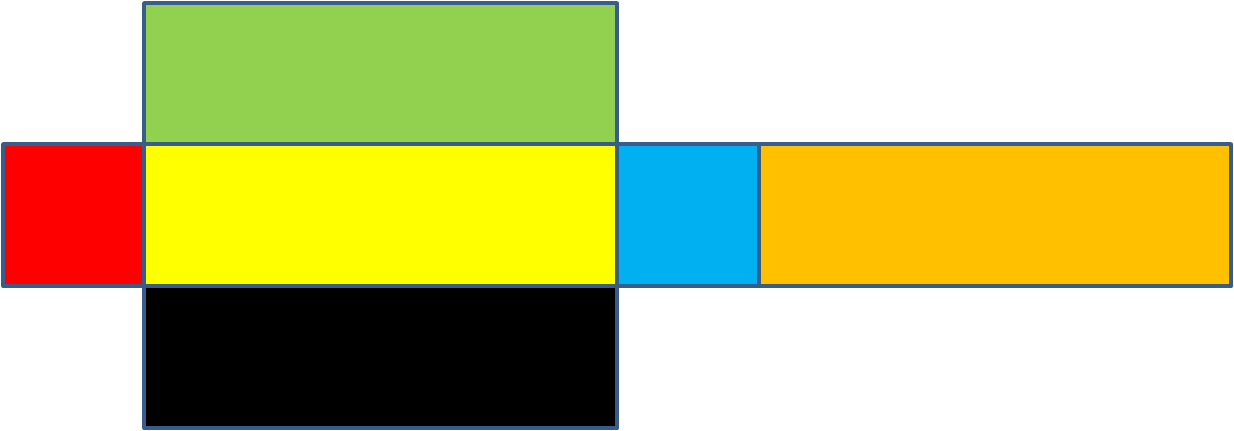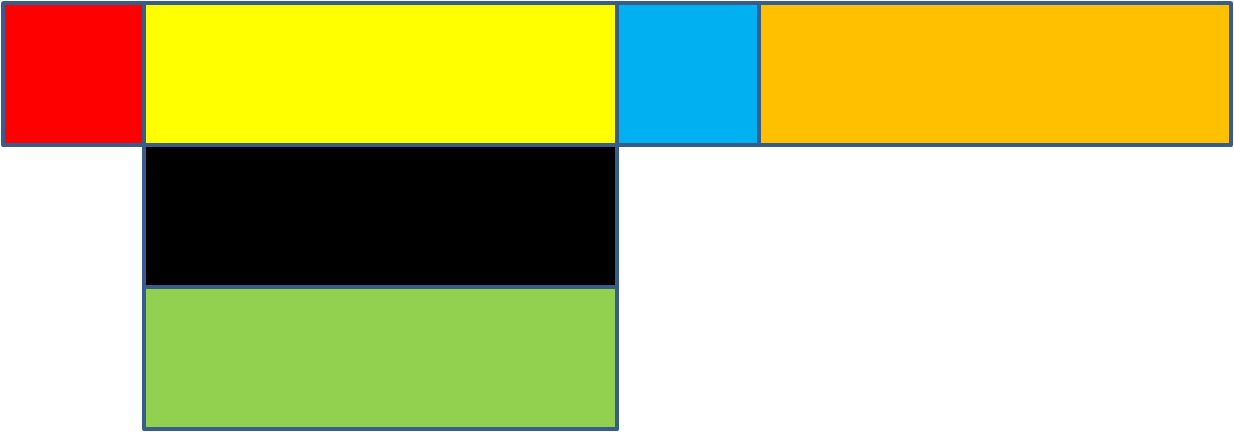# Geometry and 3D Shapes: Identifying Cuboid Nets (2)

In this worksheet, students identify nets of cuboids.Key stage:  KS 2

Curriculum topic:   Geometry: Properties of Shapes

Curriculum subtopic:   Identify 3D Shapes

Difficulty level:### QUESTION 1 of 10

The net of a 3-D solid is the shape that must be cut out of cardboard or paper

to fold into the given shape.

ExampleThis net folds into the following cuboid.True or False?

This is a net of a cuboid.True

False

True or False?

This is a net of a cuboid.True

False

True or False?

This is a net of a cuboid.True

False

True or False?

This is a net of a cuboid.True

False

True or False?

This is a net of a cuboid.True

False

True or False?

This is a net of a cuboid.True

False

True or False?

This is a net of a cuboid.True

False

True or False?

This is a net of a cuboid.True

False

True or False?

This is a net of a cuboid.True

False

True or False?

This is a net of a cuboid.True

False

• Question 1

True or False?

This is a net of a cuboid.True
EDDIE SAYS
Folded the net will have 6 faces, 8 vertices, and 12 edges, which are the properties of a cuboid.
• Question 2

True or False?

This is a net of a cuboid.True
EDDIE SAYS
Folded the net will have 6 faces, 8 vertices, and 12 edges, which are the properties of a cuboid.
• Question 3

True or False?

This is a net of a cuboid.True
EDDIE SAYS
Folded the net will have 6 faces, 8 vertices, and 12 edges, which are the properties of a cuboid.
• Question 4

True or False?

This is a net of a cuboid.False
EDDIE SAYS
This is not a cuboid because the net doesn't have a square face on both sides.
• Question 5

True or False?

This is a net of a cuboid.False
EDDIE SAYS
This is not a cuboid because the net doesn't have a square face on both sides.
• Question 6

True or False?

This is a net of a cuboid.True
EDDIE SAYS
Folded the net will have 6 faces, 8 vertices, and 12 edges, which are the properties of a cuboid.
• Question 7

True or False?

This is a net of a cuboid.False
EDDIE SAYS
This is not a cuboid net because one rectangular face is missing.
• Question 8

True or False?

This is a net of a cuboid.True
EDDIE SAYS
Folded the net will have 6 faces, 8 vertices, and 12 edges, which are the properties of a cuboid.
• Question 9

True or False?

This is a net of a cuboid.True
EDDIE SAYS
Folded the net will have 6 faces, 8 vertices, and 12 edges, which are the properties of a cuboid.
• Question 10

True or False?

This is a net of a cuboid.True
EDDIE SAYS
Folded the net will have 6 faces, 8 vertices, and 12 edges, which are the properties of a cuboid.
---- OR ----